Question

# Here is some data on the economy of a certain country. Use this data for all...

Here is some data on the economy of a certain country. Use this data for all three parts of question 1.

M1 Money Supply:   $190 Billion Real GDP:$765 Billion

Velocity of M1 Money Supply:   4.3

Question 1a: Right now, what is the rate of inflation in that country? How can you tell?

Question 1b: If they want to change the inflation rate to about 2% (which the Fed says is ideal), can they do so by changing the Money Supply? Assuming the velocity stays the same, about how much should the Money Supply be to get to the inflation rate to 2%

Question 1c: Give one example of action the Central Bank of that country could take to reduce the money supply. Explain how your example would work. For this question, assume that the Central Bank of that country has tools for Monetary Policy similar to the tools used by the F.O.M.C. in the USA. You don’t need specific numbers to answer this, just a clear explanation.

Consider the quantity theory of money supply as given in the following form -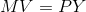Given -

M = 190 billion

V = 4.3

Y = 765 billion

We get inflation/price level as. -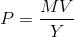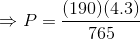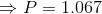If P = 2, V = 4.3 and Y = 765, we get -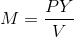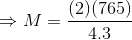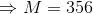One way for the Central Bank to increase the money supply in the economy is to reduce the discount rate. This is the rate at which the central bank lends money to the commercial banks. If this rate is reduced, then the commercial banks can borrow money from the central bank at a lower interest rate, which will reduce the cost of borrowing for the commercial banks, in which case they will borrow more and advance more loans to the public, which will increase the money supply in the economy.

#### Earn Coins

Coins can be redeemed for fabulous gifts.

Similar Homework Help Questions
• ### Question 1: Inflation and Monetary Policy (12 points out of 20) Here is some data on...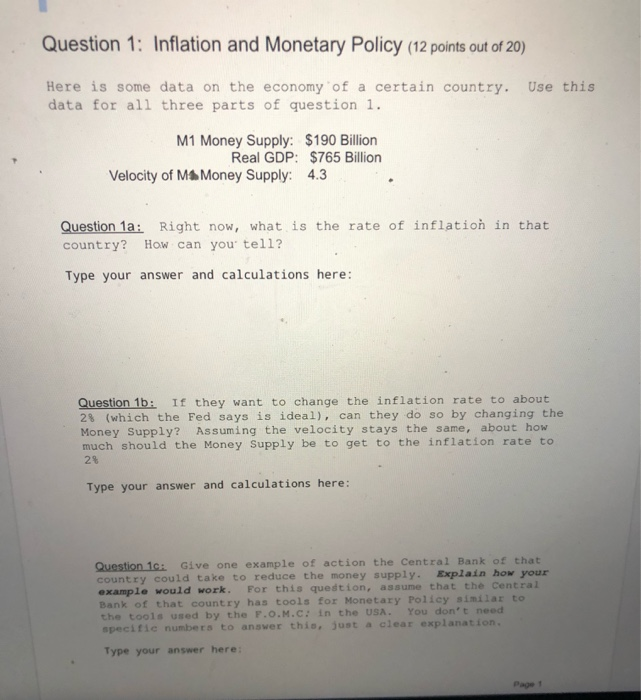Question 1: Inflation and Monetary Policy (12 points out of 20) Here is some data on the economy of a certain country. Use this data for all three parts of question 1. M1 Money Supply: $190 Billion Real GDP:$765 Billion    Velocity of M1 Money Supply: 4.3 Question 1a: Right now, what is the rate of inflation in that country? How can you tell? Type your answer and calculations here:    Question 1b: If they want to change the inflation rate...

• ### a country has no travelor checks and it has the data given below. what is the...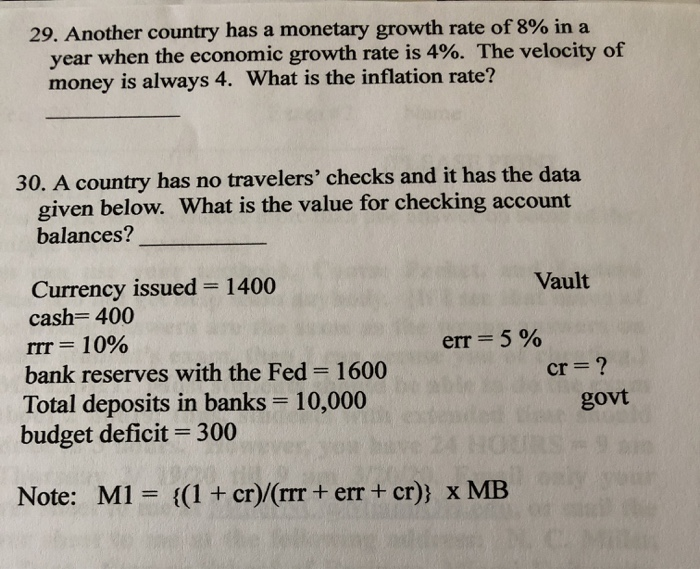a country has no travelor checks and it has the data given below. what is the value gor checking account balances. 29. Another country has a monetary growth rate of 8% in a year when the economic growth rate is 4%. The velocity of money is always 4. What is the inflation rate? 30. A country has no travelers' checks and it has the data given below. What is the value for checking account balances? Vault err = 5% Currency...

• ### Consider an economy with the following Money market information. Is the past year inflation rate was...

Consider an economy with the following Money market information. Is the past year inflation rate was 4%, the output grew by 5%, and the nominal interest rate on non-monetary assets grew by 2.5%. Moreover, we know that the central bank of this economy increased the Money supply of economy by 6%. As an economist you know that the interest elasticity of Money demand of this economy is -0.2. However, you don’t have any information about the income elasticity of Money...

• ### need all 5 answeres If the monetary base is $1,000 billion, checkable deposits are$2,000 billion,...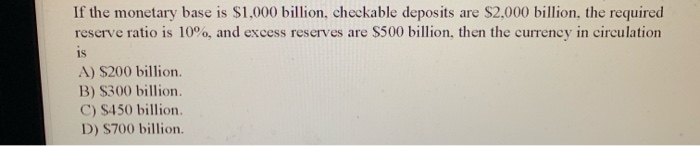need all 5 answeres If the monetary base is $1,000 billion, checkable deposits are$2,000 billion, the required reserve ratio is 10%, and excess reserves are $500 billion, then the currency in circulation are$500 billion, then 92,000 billion. A) $200 billion B)$300 billion. C) $450 billion. D)$700 billion. When the Federal Reserve wants to raise interest rates after banks have accumulated large amounts of excess reserves (i.e., when the supply curve intersects the demand curve at the...

• ### The following data represented the situation in the economy of Epsilon as of January 1, 2016....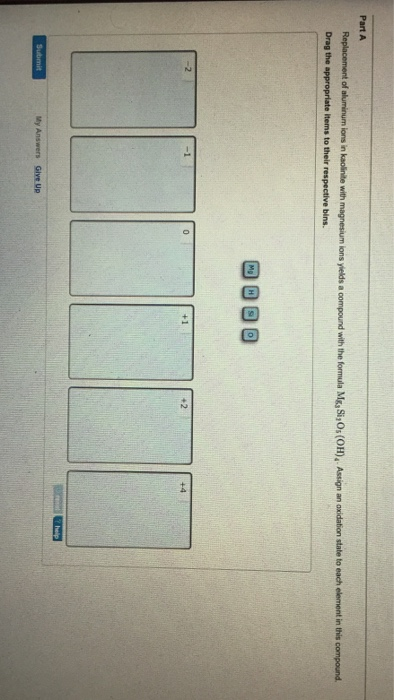The following data represented the situation in the economy of Epsilon as of January 1, 2016. O MPC = .75 Demand Deposits in the banking system = S100 billion Actual Reserves in the banking system - S20 billion Reserve Requirement, rr = .2 Current Unemployment Rate = 7.3% Current Rate of inflation 6.6% Natural Rate of Unemployment 5% Trend Rate of Inflation = 2% Use an aggregate demand/aggregate supply graph to show and explain the equilibrium in Epsilon as of...

• ### really money supply, monetary base, nominal GDP, real GDPprice level and M1 21. If the capital...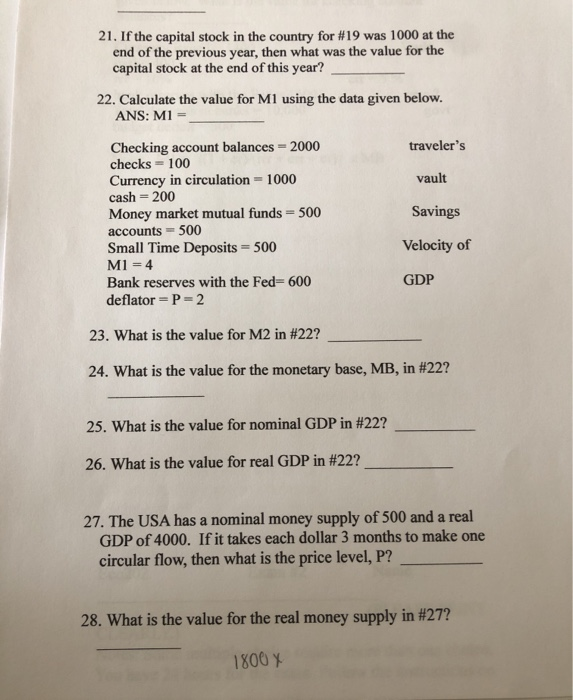really money supply, monetary base, nominal GDP, real GDPprice level and M1 21. If the capital stock in the country for #19 was 1000 at the end of the previous year, then what was the value for the capital stock at the end of this year? 22. Calculate the value for M1 using the data given below. ANS: M1 = traveler's vault Savings Checking account balances = 2000 checks = 100 Currency in circulation - 1000 cash = 200 Money...